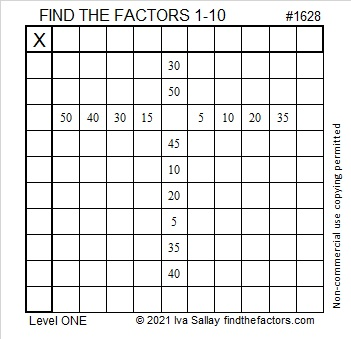# 1628 A Simple Cross

Contents

### Today’s Puzzle:

A simple cross is an appropriate symbol for Good Friday. Write the numbers from 1 to 10 in the first column and the top row so that those numbers and the given clues function like a multiplication table.### Factors of 1628:

• 1628 is a composite number.
• Prime factorization: 1628 = 2 × 2 × 11 × 37, which can be written 1628 = 2² × 11 × 37.
• 1628 has at least one exponent greater than 1 in its prime factorization so √1628 can be simplified. Taking the factor pair from the factor pair table below with the largest square number factor, we get √1628 = (√4)(√407) = 2√407.
• The exponents in the prime factorization are 2, 1, and 1. Adding one to each exponent and multiplying we get (2 + 1)(1 + 1)(1 + 1) = 3 × 2 × 2 = 12. Therefore 1628 has exactly 12 factors.
• The factors of 1628 are outlined with their factor pair partners in the graphic below.### More about the Number 1628:

1628 is the hypotenuse of a Pythagorean triple:
528-1540-1628, which is (12-35-37) times 44.

1628 is the difference of two squares in two different ways:
408² – 406² = 1628, and
48² – 26²  = 1628.

This site uses Akismet to reduce spam. Learn how your comment data is processed.